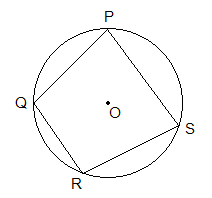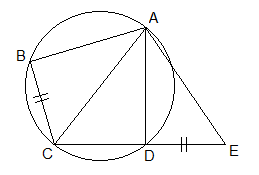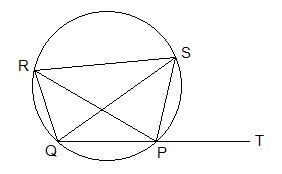A quadrilateral having its all four vertices on the circumference of a circle is called a cyclic quadrilateral.

********************

10 Math Problems officially announces the release of Quick Math Solver, an Android App on the Google Play Store for students around the world.

********************ABCD, in the given figure, is a cyclic quadrilateral.

### Concyclic Points

The points lying on the circumference of the circle are called concyclic points.In the given circle, A, B, C, D are concyclic points.### Theorems on Cyclic Quadrilateral and Their Proofs:

THEOREM 1:

The sum of opposite angles of a cyclic quadrilateral is always 180°.

PROOFS:Given: PQRS is a cyclic quadrilateral.

To Prove:    (i) QPS + QRS = 180°

(ii) PQR + PSR = 180°

Proof:

Statements                         Reasons

1.     QPS = ½ Arc QRS -----> Inscribed angle is equal to the half of its opposite arc.

2.     QRS = ½ Arc QPS -----> Inscribed angle is equal to half of its opposite arc.

3.     QPS + QRS = ½ Arc QRS + ½ Arc QPS -----> Adding statements 1 and 2.

or, QPS + QRS = ½ Arc (QRS + QPS)

or, QPS + QRS = ½ Circle PQRS

or, QPS + QRS = ½ 360°

or, QPS + QRS = 180°

4.     PQR + PSR = 180° -----> Same as above.

Hence proved.

THEOREM 2:

In a cyclic quadrilateral, the exterior angle is equal to its opposite interior angle.

PROOFS:Given: ABCD is a cyclic quadrilateral with exterior angle DCE.

Proof:

Statements                         Reasons

1.     DCE + BCD = 180° -----> Opposite angles of a cyclic quadrilateral are supplementary.

2.     BAD + BCD = 180° -----> Linear pair of angles.

3.     DCE + BCD = BAD + BCD -----> From statements 1 and 2.

4.     DCE = BAD -----> Cancelling common angle BCD.

Hence proved.

#### Worked Out Examples

Example 1: Find COD in the given figure.Solution: From the figure,

110° + ODC = 180° [Opposite angles of a cyclic quadrilateral]

or,     ODC = 180° – 110°

or,     ODC = 70°

ODC = OCD = 70° [Being OC = OD, radii of same circle]

COD + ODC + OCD = 180° [Sum of angles of DCOD]

or,     COD + 70° + 70° = 180°

or,     COD + 140° = 180°

or,     COD = 180° – 140°

or,     COD = 40°  Ans.

Example 2: Calculate the value of x and y in the given figure.Solution: From the figure,

x = ½ BOD [Inscribed angle is half of central angle]

or,     x = ½ 160°

or,     x = 80°

y + x = 180° [Opposite angles of a cyclic quadrilateral]

or,     y + 80° = 180°

or,     y = 180° – 80°

or,     y = 100°

Hence, x = 80° and y = 100°  Ans.

Example 3: Find the angle x in the given figure.Solution: From the figure,

CAD = 56° [Inscribed angle on same arc CD]

Now,

= 96° – 56°

= 40°  Ans.

Example 4: In the adjoining figure, ABCD is a cyclic quadrilateral, side CD is produced to the point E, where BC = DE. If CA bisects BCD prove that DACE is an isosceles triangle.

Solution: Here,Given: ABCD is a cyclic quadrilateral. BC = DE and BCA = ACD.

To Prove: DACE is an isosceles triangle.

Proof:

Statements                Reasons

1.     BCA = ACD -----> Given.

2.     Arc AB = arc AD -----> Arcs subtended by equal inscribed angles.

3.     AB =AD ------> Equal chords cut off equal arcs in a circle.

i.       AB = AD (S) -----> From statement 3

ii.    ABC = ADE (A) -----> Exterior angle of a cyclic quadrilateral

iii. BC = DE (S) -----> Given.

5.     DABC DADE -----> By SAS axiom

6.     AC = AE -----> Corresponding sides of triangles.

7.     ACE is an isosceles triangle -----> Being AC = AE.

Hence proved.

Example 5: In the given figure, SP is the bisector of RPT and PQRS is a cyclic quadrilateral. Prove that SQ = SR.

Solution: Here,Given: PQRS is a cyclic quadrilateral. RPS = SPT.

To Prove: SQ = SR.

Proof:

Statements               Reasons

1.     SPT = RPS -----> From given.

2.     SPT = QRS -----> Exterior angle of cyclic quadrilateral PQRS.

3.     SQR = RPS -----> Inscribed angle on same arc  RS.

4.     SQR = QRS -----> From statements 1, 2 and 3.

5.     SQ = SR -----> Being base angles of DSQR equal, statement 4.

Hence proved.

If you have any question or problems regarding the Cyclic Quadrilaterals, you can ask here, in the comment section below.